### Gravitational Physics

To talk about gravity, we need a solid conception of mass itself. In Newtonian gravity, mass appears in two central roles, which we will discern in this quiz:

• The weight of an object is directly proportional to its mass $$m$$.
• In Newton's second law, an object's mass $$m$$ controls its response to forces.

When we are calculating the effects of gravity on a falling object, mass takes on both roles simultaneously. In no other classical theory is one quantity endowed with two interpretations; in fact the mysterious dual role of mass is the point of departure for Einstein’s celebrated theory of gravity, general relativity.

Without further ado, let’s begin our exploration of gravity with the mystery of the white room.# Explore: What is Mass?

Your eyes blink open in an intensely white room. Beside you lay two cubes, one white and one black. You have no idea how you got here. You shakily lift yourself from a supine position.A syrupy voice informs you that you have fallen into a sensory test chamber. Beside you, one cube is real matter and the other is a sensory distortion.

"What?" you yell, but there is no response.

You examine the cubes, picking up one in each hand. They both feel pretty real to you.

# Explore: What is Mass?

Suddenly, the voice says menacingly: "This test will end only if you can name the cube that is not real. You get one try."

Startled, you drop both cubes.Both cubes fall from the same height with identical initial speeds. They do not hit the ground at the same time. Are their accelerations identical or different?

# Explore: What is Mass?

Gravity is the force that causes objects to fall on Earth's surface, and is the subject of this course. Gravity's action is a major consideration in how we choose to manipulate the objects around us; it is also responsible for sculpting our solar system and the larger universe. Progressing through this course, you will examine gravity's effects on successively larger scales, and learn about gravity's many faces.

Gravity always acts between pairs of objects. In the white room, gravity is acting between the Earth and each block as it falls.

The acceleration due to gravity depends only on one mass in the pair. Which one?

# Explore: What is Mass?

In Newton's theory of gravity, Earth's mass generates a gravitational field $$\mathbf{g}$$ that pulls the cubes toward its center. At the surface, Earth's gravitational field has a magnitude $$g=\SI[per-mode=symbol]{9.8}{\meter\per\second\squared}.$$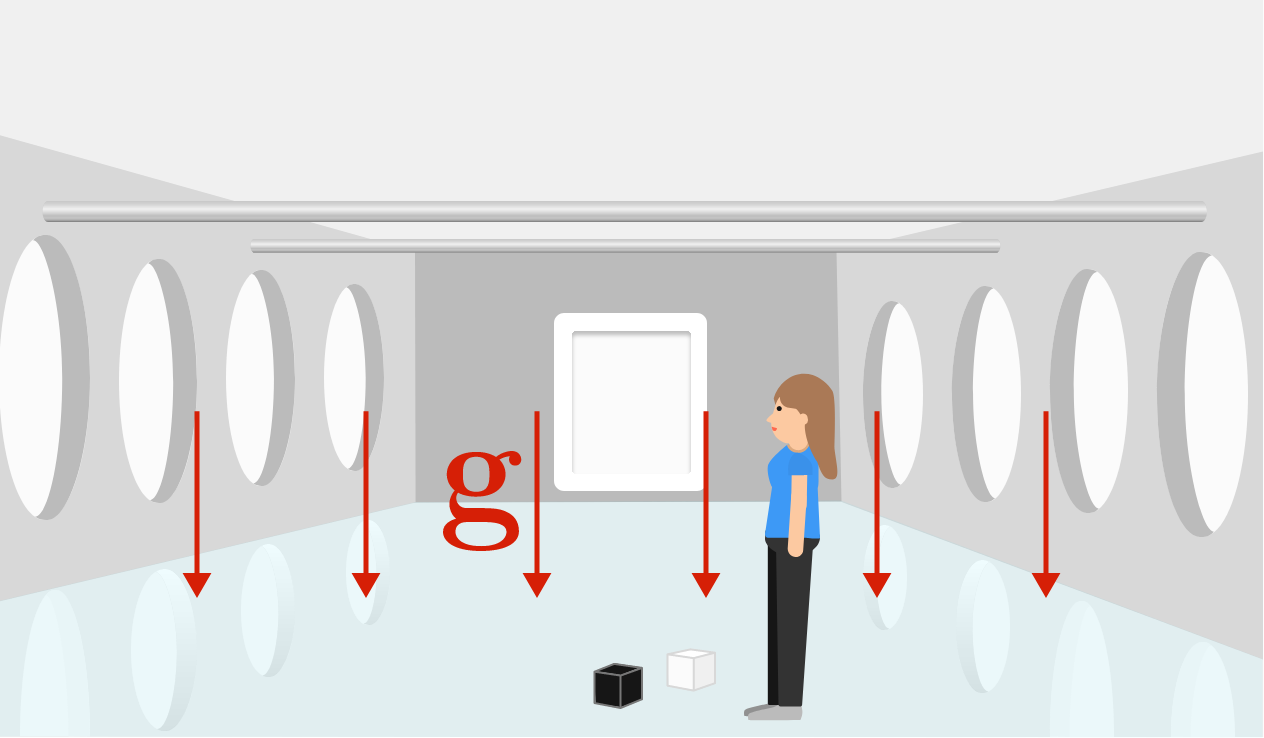The gravitational field has the same units as acceleration because, as we saw in the previous question, any mass in free-fall has the same acceleration.

What force $$F_g$$ would produce this acceleration on a mass $$m$$ during free-fall near Earth's surface?

Hint: Use Newton's second law.

# Explore: What is Mass?

To begin testing for differences between the cubes, you attach a string (from your pocket) to both cubes, and hang them over one of the horizontal supports above you to compare their weights.

It turns out they balance; neither one accelerates when you let go.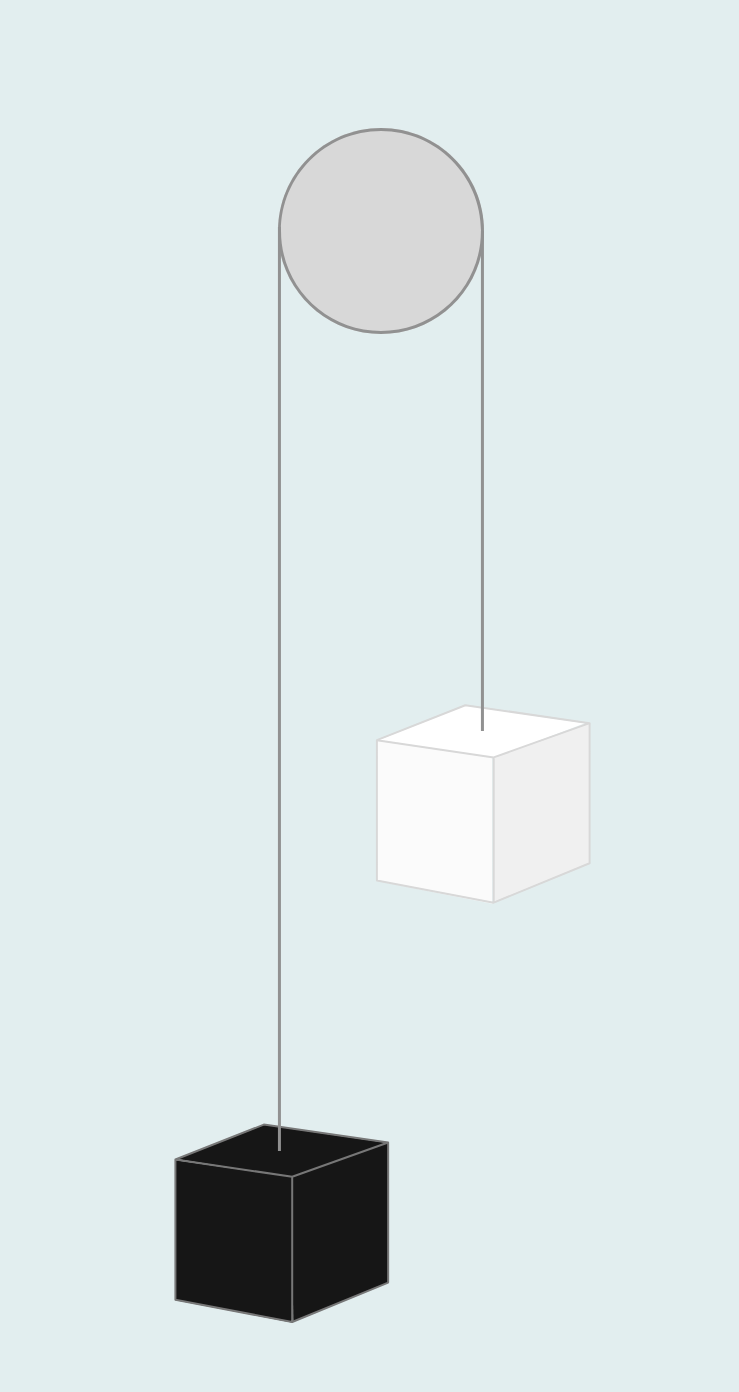Which cube weighs more?

Details & Assumptions

• There is negligible friction between the string and the support.
• The string weighs much less than either cube, so you can neglect its weight.

# Explore: What is Mass?

Before, you observed a difference between the cubes while they were moving, so you decide to tie the string tightly around the horizontal support. The cubes can now swing like pendulums.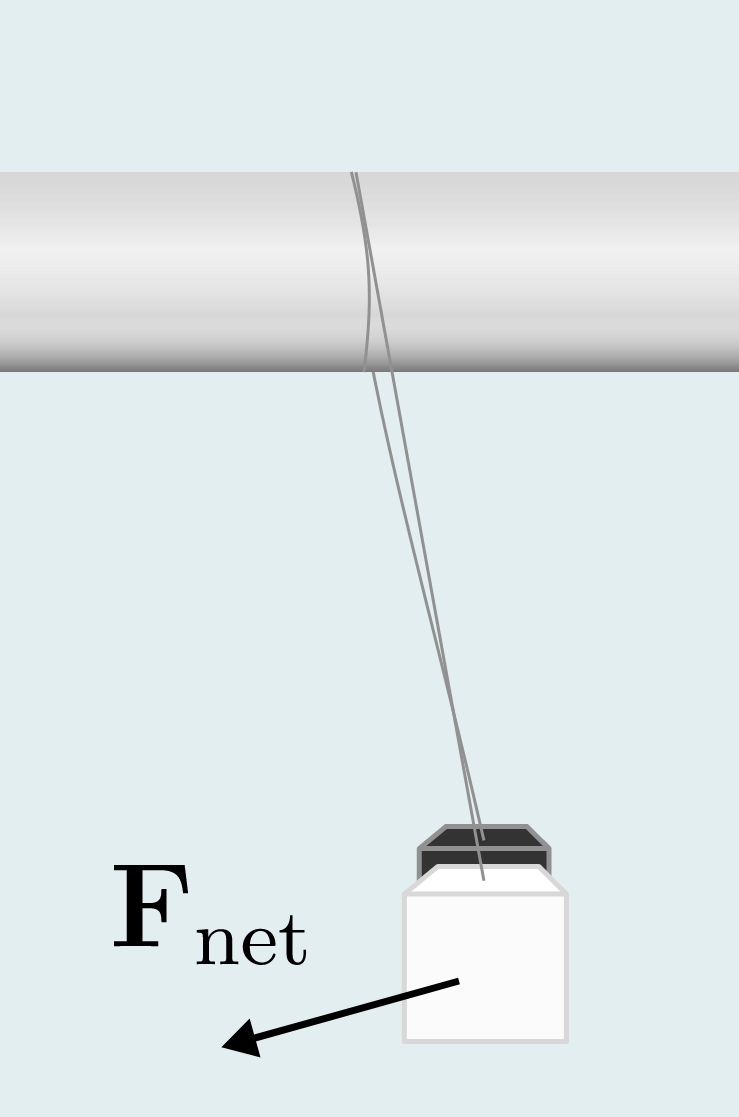You may recall that this oscillation results from an imbalance between weight (which you already deduced is the same for both blocks) and a tension force acting along the string.

If you pull both blocks back to the same height and release them, which block feels a larger net force $$F_\text{net}$$ the instant you let go?

# Explore: What is Mass?

After you let go, the cubes swing back and forth (without rotating or bumping each other) and you notice that the white cube has a distinctly longer oscillation period.

Thus, one of the cubes has larger maximum acceleration than the other. Which one?

Details & Assumptions

• Assume the motion of both blocks is simple harmonic, with angular frequency $$\omega$$ and the period $$T$$ related by $$\omega=2\pi/T.$$
• The cubes are released from the same height and the strings connecting them to the support have the same length.

# Explore: What is Mass?

Both cubes have the same weight in Earth's gravitational field, yet they have different accelerations under the same net force. One of the cubes is violating the ordinary Newtonian physical rules, but which rule?

In our testing, we have identified two roles of an object's mass $$m$$:

1. determining the magnitude of the gravitational force, as in $$F_g = mg.$$
2. representing an object's inertia in Newton's second law, as in $$F=ma$$.

The equivalence principle asserts that an object's gravitational mass is identical to its inertial mass. This is an often-overlooked postulate of Newtonian physics derived from observation. Perhaps surprisingly, it is not derivable from Newton's laws!

# Explore: What is Mass?

You cannot identify which cube is real without comparing the cubes to another object with a known physical existence.

Fortunately, in your pocket, you carry a lucky (and very real) coin. You lay it on top of the black cube, then you drop the cube. The cube and the coin do not fall together.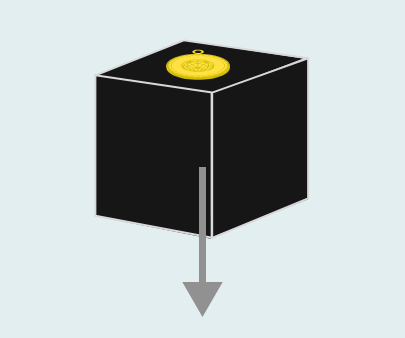Which cube is real?

# Explore: What is Mass?

You call out your deduction, and the door opens flooding the room with red light. You slowly walk across the threshold.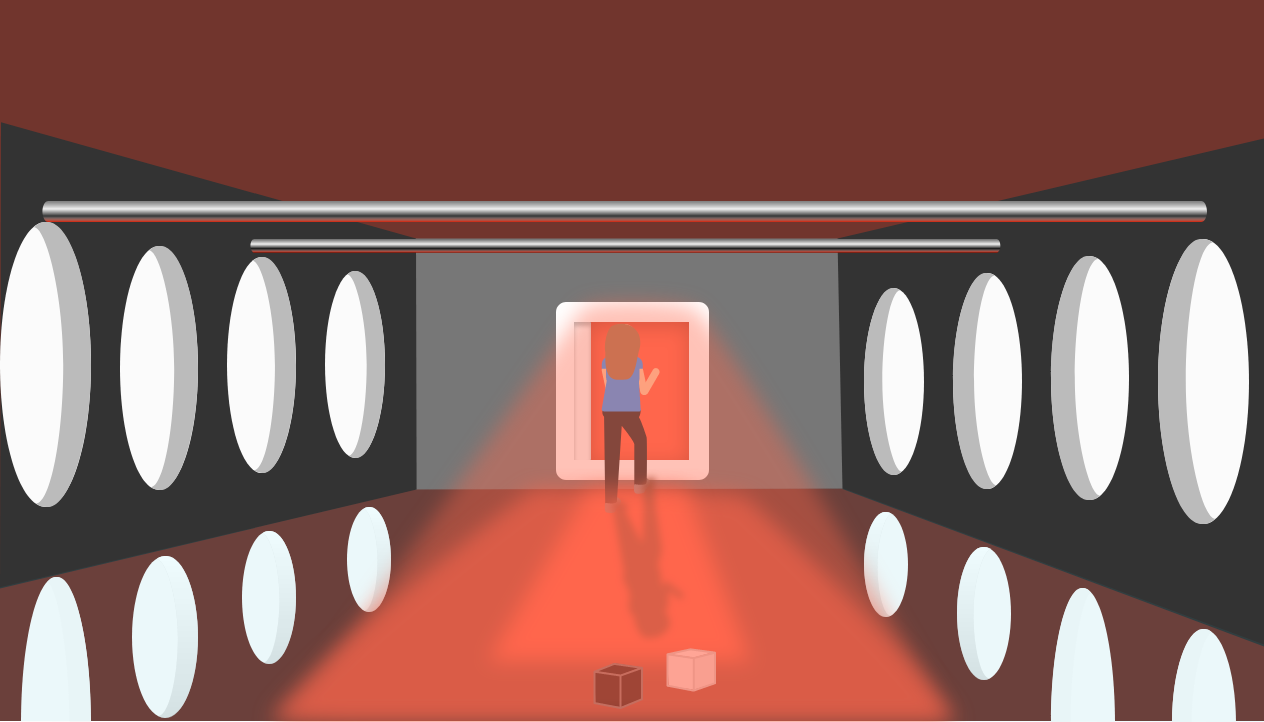Gravity's grip extends far beyond Earth's surface. It pulses through the emptiness of space, sculpting unfathomably large galaxies around objects so massive that light cannot escape from them. Mass is both the source and the subject of gravity's action. How mass is distributed throughout the universe is inexplicable without understanding gravity's action.

This course is your portal to understanding gravity's far-reaching effects. The focus of this first chapter is to introduce Newton's revolutionary idea of a universal force between masses.

# Explore: What is Mass?

×